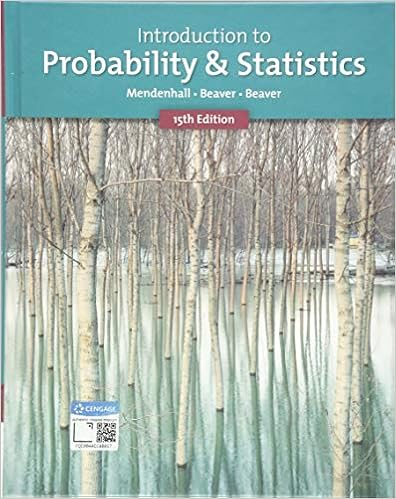# Probability and Statistics for Engineering and the Sciences (with CD-ROM and InfoTrac )

• Homework Help
• PresidentHackerCaribou10582
• 2

This preview shows page 2 out of 2 pages.

##### We have textbook solutions for you!
The document you are viewing contains questions related to this textbook.The document you are viewing contains questions related to this textbook.
Chapter 9 / Exercise 4
Introduction to Probability and Statistics
Beaver/MendenhallExpert Verified
Since the P -value is larger than 0.3, we do not re- ject H 0 at level 0.3. (d) Although qnorm values are not given, we can estimate z 0 . 32 / 2 from the R output. Note that P ( Z < z 0 . 32 / 2 ) = 1 - P ( Z > z 0 . 32 / 2 ) = 1 - 0 . 32 / 2 = 0 . 84 .S So z 0 . 32 / 2 = 1 . A 100(1 - α )% confidence interval is ˆ p 1 - ˆ p 2 ± z α/ 2 p ˆ p 1 (1 - ˆ p 1 ) / 100 + ˆ p 2 (1 - ˆ p 2 ) / 110 . With ˆ p 1 = 40 / 100 and ˆ p 2 = 50 / 100 , we get [ - 0 . 12 , 0 . 01] . 4. Bonus doggy problem: name the title of a movie where there is a dog carrying a human body part in its mouth(1 point). Solution. Akira Kurosawa’s Yojimbo. He is a Japanese movie director. Many of his Samurai movies had influenced Hollywood western movies. Self study problem: Compare Kurosawa’s Yojimbo (1961) and Clint Eastwood’s spaghetti western A Fistful of Dollars (1964). In Yojimbo, there is a somewhat morbid but funny scene about a dog carrying a human hand in its mouth at the beginning of the movie. 2
##### We have textbook solutions for you!
The document you are viewing contains questions related to this textbook.The document you are viewing contains questions related to this textbook.
Chapter 9 / Exercise 4
Introduction to Probability and Statistics
Beaver/MendenhallExpert Verified
•••